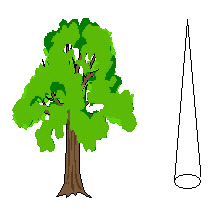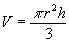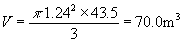# Forestry: Estimating Volumes of TreesI have a number of trees in a stand and I want to estimate the volume of the trees. One tree in particular is 43.5m high, and the dbh (diameter at basal height) is 2.48 m. The dbh is the diameter of the tree at a height of about 1-2m above the ground. Below this point the tree trunk really spreads out and is ignored.

## Solution:

Most of the valuable wood is in the trunk of the tree. The shape of the trunk can be approximated by a cone whose height is that of the tree, and whose basal diameter is the dbh of the tree.

### Setting up the problem - translating from words to symbols:

Let h be the height of the cone and r be its radius at the base. The formula for the volume of the cone is### Calculations:

The radius is half the diameter, or r = 2.48/2 = 1.24 m and h = 43.5 m. If we substitute these numbers into the equation for the volume, we getHence the estimated volume of the tree is 70.0 m3Written by G. John Smith, September 16, 1997